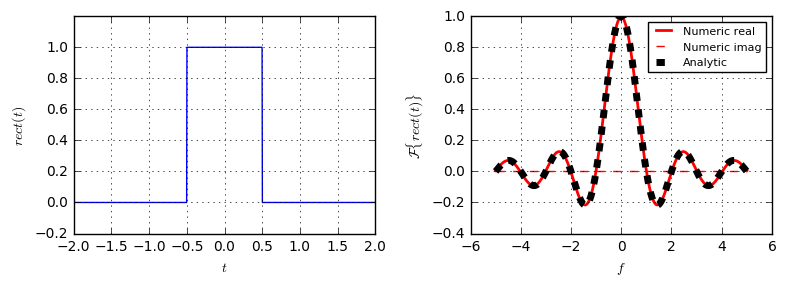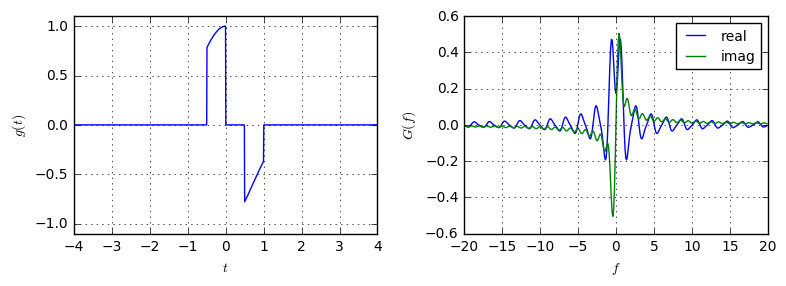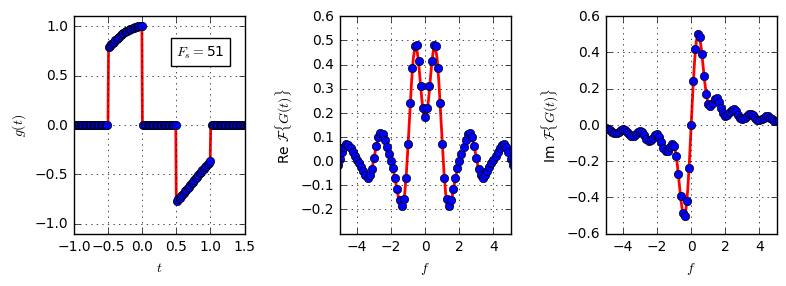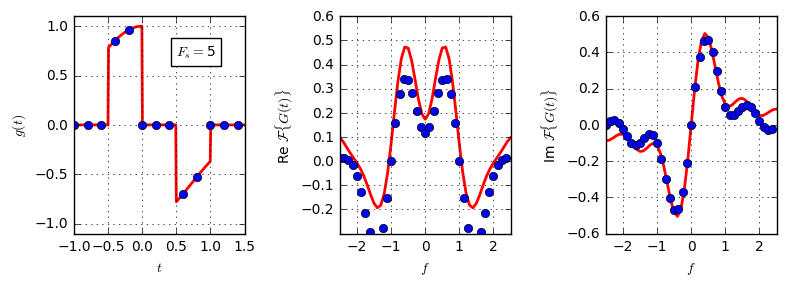## Using the Discrete Fourier Transform to approximate the Continuous-Time Fourier Transform¶

In this article, we want to understand, how we can employ the discrete Fourier transform to approximate the continuous-time Fourier Transform. We are particularly interested, because there exist fast implementations of the DFT (see Fast-Fourier-Transform which make the calculations much quicker and easier to accomplish. Furthermore, in the world of digital signal processing, most signals are discrete, making the DFT a very suitable tool.

Let us remember the Fourier Integral for a time-limited function $g(t)$ with $g(t)=0, |t|>t_0$. It is given by

\begin{equation} G(f)=\int_{-t_0}^{t_0} g(t) \exp(-j2\pi ft)dt, \end{equation}

where the integration limits are chosen because $g(t)=0$ outside of this interval. In the following, we will derive how this integral can be approximated by the discrete Fourier transform.

To confirm our results, let us first define the Python function cft, which numerically evaluates this integral by means of the Simpsons Integration rule:

def cft(g, f):
"""Numerically evaluate the Fourier Transform of g for the given frequencies"""
result = np.zeros(len(f), dtype=complex)

# Loop over all frequencies and calculate integral value
for i, ff in enumerate(f):
# Evaluate the Fourier Integral for a single frequency ff,
# assuming the function is time-limited to abs(t)<5
result[i] = complex_quad(lambda t: g(t)*np.exp(-2j*np.pi*ff*t), -5, 5)
return result

"""Return definite integral of complex-valued g from a to b,
using Simpson's rule"""
# 2501: Amount of used samples for the trapezoidal rule
t = np.linspace(a, b, 2501)
x = g(t)
return integrate.simps(y=x, x=t)


Let us assert the correctness of this function by checking the Fourier Transform of the rectangular window, where we know the correspondence

$$\mathcal{F}\{rect(t)\}=\frac{\sin(\pi f)}{\pi f}.$$
def rect(t):
return (abs(t) < 0.5).astype(float)

t = np.linspace(-2,2, 1000)

plt.subplot(121)
plt.plot(t, rect(t))

f = np.linspace(-5, 5, 1000)
R = cft(rect, f)  # Calculate the numeric Fourier Transform of the rect function

plt.subplot(122)
plt.plot(f, R.real, 'r-', lw=2, label='Numeric real')
plt.plot(f, R.imag, 'r--', label='Numeric imag')
plt.plot(f, np.sin(np.pi*f)/(np.pi*f), 'k--', lw=5, label='Analytic')Now, remember that a (Riemann) integral such as the Fourier integral can be understood as the sum of infinitely many small rectangles, that approximate the curve in the integral. Let us divide the interval of width $2t_0$, ranging from $-t_0$ to $t_0$, into $N$ equally spaced sub-intervals. Hence, these sub-intervals have a width of $dt=\frac{2t_0}{N}$. This procedure is illustrated in the following code, where we use a parabola as the function to integrate. Analytically, we know: $$\int_{-\frac{1}{2}}^{\frac{1}{2}}1-4t^2dt=\frac{2}{3}$$

def g(t):
return (1-4*t*t) * abs(np.array(t)<=0.5).astype(float)

t0 = 0.5
t = np.linspace(-t0, t0, 1000)

def riemannApprox(N):
dt = 2*t0/N
n = np.arange(N)
plt.plot(t, g(t))
plt.bar(n*dt-t0, g(n*dt-t0), width=dt, color='#eeeeff')
text = ("Points: %d\n" % N +
"Correct Integral: %.4f\n" % integrate.quad(g, -t0, t0) +
"Approximated Integral: %.4f" % (dt * sum(g(n*dt-t0))))
plt.text(-0.0, 0.2, text, ha='center',bbox=dict(facecolor='white'))




As we see, the result from the approximation becomes closer to the correct value, the more points are used for the approximation.

By using this Riemann-sum approximation, we can approximate the Fourier integral by

$$G(f) \approx \sum_{n=0}^{N-1}g(n\cdot dt-t_0) \exp(-j2\pi f(n\cdot dt -t_0))dt$$

Now, note that by dividing the time range into small intervals and assinging a constant value of the signal for each interval, we essentially simply perform time-sampling of the signal and the sampling frequency is given by $F_s=\frac{N}{2t_0}=\frac{1}{dt}$ (i.e. every $\frac{1}{F_s}$ seconds there is a new sample, yielding N samples in the time range of $2t_0$.). We now substitute $dt$ into the integral approximation to get

$$G(f)\approx \frac{1}{F_s}\sum_{n=0}^{N-1}g(\frac{n}{F_s}-t_0) \exp(-j2\pi \frac{fn}{F_s})\exp(j2\pi ft_0).$$

Let us define the sampled version of $g$ by

$$g[n]:=g(\frac{n}{F_s}-t_0).$$

We can now compare the expression for the approximate integral with the expression for the discrete Fourier Transform of the signal:

$$G[k] = \sum_{n=0}^{N-1}g[n]\exp(-j2\pi\frac{kn}{N}).$$

We already see a close similarity between both expressions. Finally, identifying that the DFT calculates the Fourier Transform only for some discrete frequencies which are given by $f=\frac{k}{N}F_s$, we get to the final expression

$$G(f=\frac{k}{N}F_s) \approx \frac{1}{F_s}\exp(j2\pi ft_0)G[k].$$

Hence, we can approximate the continuous-time Fourier transform of a time-limited signal by the Discrete Fourier Transform. The extra phase shift factor $\exp(j2\pi ft_0)$ amounts to the fact, that the continuous-time integration already starts at time $t=-t_0$, wheres the DFT assumes starting at time $t=0$.

The approximation by the DFT holds the better, the higher the sampling frequency (i.e. the number of samples in the interval) is. Also note, that, according to the Nyquist sampling theorem, the sampling frequency should be at least twice the bandwidth of the signal.

The careful student might have found a contradiction in the last statement: For our approximation to work, we require the signal to be time-limited. At the same time, the DFT only approximates correctly, if the sampling frequency is at least twice the bandwidth. However, according to the uncertainty principle of the Fourier Transform no time-limited signal can be also band-limited. As such, the approximation can never be exactly correct. However in practive, we just need to take care that the sampling frequency is high enough to contain most of the energy of the signal.

Let us verify our findings numerically. First, let's define some function and calculate its continuous-time Fourier Transform. To make it more interesting, our function is the sum of two rectangular functions which is multiplied with some Gaussian. Note that, in a strict sense, this function is not band-limited since it consists of rectangular functions which have an unlimited spectrum. Hence, the DFT can only approximate the spectrum, since we cannot guarantee sampling at the Nyquist rate. We will see below what happens, when the sampling rate is too low.

g = lambda t: (rect(t) - rect(t-0.5)) * np.exp(-t*t)  # define the function
t0 = 4
f_full = np.arange(-20, 20, 0.1)
t_full = np.linspace(-t0, t0, 1000)
G_exact = cft(g, f_full)  # calculate Fourier Transform of the function

plt.subplot(121)
plt.plot(t_full, g(t_full))

plt.subplot(122)
plt.plot(f_full, G_exact.real, label='real')
plt.plot(f_full, G_exact.imag, label='imag')Now, lets plot the exact Fourier Transform and the approximation from the DFT on top of each other. First, define a Python function compareFTandDFT that takes the sampling frequency and then plots the exact FT and the approximation by the DFT:

def compareFTandDFT(Fs):
t = np.arange(-t0, t0, 1./Fs)
f = np.linspace(-Fs/2, Fs/2, len(t), endpoint=False)
# Calculate the approximate Fourier Transform according to the derived rule.
G_approx = np.fft.fftshift(np.fft.fft(g(t)) * np.exp(-2j*np.pi*f*t0) * 1/Fs)

plt.subplot(131)
plt.plot(t_full, g(t_full), 'r-', lw=2)
plt.plot(t, g(t), 'o')

plt.subplot(132)
plt.plot(f_full, np.real(G_exact), 'r-', lw=2)
plt.plot(f, np.real(G_approx), 'o')

plt.subplot(133)
plt.plot(f_full, np.imag(G_exact), 'r-', lw=2)
plt.plot(f, np.imag(G_approx), 'o')



Let's plot the approximation for a high sampling frequency. As shown, the approximation and the exact curve match well (i.e. blue dots and red line are on top of each other).

compareFTandDFT(Fs=51)Now, let's see what happens, when the sampling frequency is very low. In this case, we do not have enough samples in the time domain to sufficiently approximate signal. Accordingly, there is a great deviation between the exact and the approximated spectrum.

compareFTandDFT(Fs=5)With our findings, we can now define a function that approximates the continuous-time Fourier Transform by means of a sampled version of the signal:

def ft(samples, Fs, t0):
"""Approximate the Fourier Transform of a time-limited
signal by means of the discrete Fourier Transform.

samples: signal values sampled at the positions t0 + n/Fs
Fs: Sampling frequency of the signal
t0: starting time of the sampling of the signal
"""
f = np.linspace(-Fs/2, Fs/2, len(samples), endpoint=False)
return np.fft.fftshift(np.fft.fft(samples)/Fs * np.exp(-2j*np.pi*f*t0))


### Conclusion¶

The Fourier Transform is a tool of very high importance in all signal processing tasks. In this article, we have derived, how the continuous-time Fourier Transform can be approximated by the discrete Fourier Transform of a sampled version of the signal.

Assume a function $g(t)$ and its spectrum $G(f)=\mathcal{F}\{g(t)\}$. Let $g[n]=g(\frac{n}{F_s}-t_0), n=0,\dots,N-1$ be a sampled version of $g(t)$, sampled at frequency $F_s$. Then, we can approximate $$G(f=\frac{k}{N}F_s) \approx \frac{1}{F_s}\exp(j2\pi ft_0)G[k],$$ where $G[k]$ is the N-Point DFT of $g[n]$.

We have also shown that the accuracy of this approximation depends on the sampling frequency, due to the Nyquist-Sampling Theorem.Do you have questions or comments? Let's dicuss below!

### Related posts

DSPIllustrations.com is a participant in the Amazon Services LLC Associates Program, an affiliate advertising program designed to provide a means for sites to earn advertising fees by advertising and linking to amazon.com, amazon.de, amazon.co.uk, amazon.it.